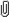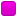September 25, 2023, 04:04:43 am

###AuthorTopic: Need help with finding coefficients of binomial expansions!  (Read 2793 times) Tweet Share

0 Members and 1 Guest are viewing this topic.

#### mwuah

• Fresh Poster
•• Posts: 1
• Respect: 0##### Need help with finding coefficients of binomial expansions!
« on: October 23, 2021, 07:49:00 pm »
0
I didn't find these questions difficult until exponents started being introduced in the brackets. Please help thanks

#### RuiAce

• ATAR Notes Lecturer
• Honorary Moderator
• Great Wonder of ATAR Notes
••• Posts: 8814
• "All models are wrong, but some are useful."
• Respect: +2575##### Re: Need help with finding coefficients of binomial expansions!
« Reply #1 on: October 23, 2021, 09:25:25 pm »
+5
I didn't find these questions difficult until exponents started being introduced in the brackets. Please help thanks

You can nevertheless write out the general term for each individual bracket.
$\text{The general term in }(3-2x)^2\text{ is }\binom{2}{j}3^{2-j}(2x)^j = \binom{2}{j} 3^{2-j}(-2)^j x^j.\\ \text{The general term in } \left( x + \frac2x\right)^5\text{ is }\binom{5}{k} x^{5-k} \left(\frac{2}{x} \right)^{k} = \binom{5}{k} 2^k x^{5-2k}.$
Different letters used for convenience. Note also that $0\leq j\leq 2$ and $0\leq k\leq 5$.
$\text{Therefore, all terms in the expansion of }(3-2x)^5 \left( x+\frac2x\right)^5\text{ will be of the form}\\ \binom{2}{j} 3^{2-j}(-2)^j \binom{5}{k} 2^k x^{j+5-2k}.$
We want all the terms such that the power on $x$ is $0$. This is satisfied whenever
$j+5-2k=0 \implies j=2k-5.$
The only values of $j$ and $k$ that satisfy this (given domain restrictions) is $j=1$ and $k=3$. Therefore the required coefficient will be the single term
$\binom{2}{1} 3^{2-1}(-2)^1 \binom{5}{3} 2^3=-960.$
Of course, if there were other values of $j$ and $k$ that did the job, you would have to add all of those individual coefficients together to get the final coefficient.

Note that it is not beneficial to your learning to spoonfeed solutions to every question. If you require guidance with the other one, you should provide appropriate working out showing what progress you made. (Doesn't have to follow the exact same working layout as the one I used above.)
Easiest way if you are unsure is to expand out the brackets and find the corresponding value.
Often not advised, since in the HSC the powers can skyrocket (well into the 20s), or are just for an unknown power $n$. (For both terms, not just one of them.)